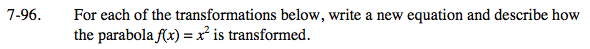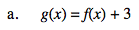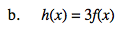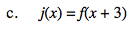Home > INT3 > Chapter 7 > Lesson 7.2.4 > Problem7-96

7-96.
1. For each of the transformations below, write a new equation and describe how the parabola f(x) = x2 is transformed. Homework Help ✎

1. g(x) = f(x) + 3

2. h(x) = 3f(x)

3. j(x) = f(x + 3)Change f(x) to x2 in the equation.

$g(x)= x^2 + 3$

Translated up 3 units.$h(x)=3x^2$